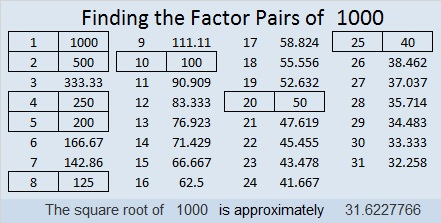# 1000 Find the Factors Challenge

Dan Bach asked me if I had anything special planned for my 1000 post. I had a few things planned, but this Find the Factors Challenge exists because of his question and our twitter conversation. I like this puzzle better than my previous plans. It is only fitting that I dedicate this puzzle to Dan Bach.

There are four places outlined in bold that need you to place the numbers 1 – 10 so that each section of the puzzle will work as a multiplication table. Each section of the puzzle is dependent on the other sections. None of the sections could be a puzzle independent of the others. You will use similar logic to solve this puzzle as you would a regular Find the Factors puzzle. It will not be easy to solve at all, but give it a try. There is only one solution.Print the puzzles or type the solution in this updated excel file: 12 factors 993-1001

1000 is a perfect cube as well as the product of perfect cubes.

30² + 10² = 1000
26² + 18² = 1000

1000 is the hypotenuse of three Pythagorean triples:
600² + 800² = 1000²
352² + 936² = 1000²
280² + 960² = 1000²

1000 looks interesting in a few bases:
2626 in BASE 7
1331 in BASE 9
1A1 in BASE 27 (A is 10 base 10)

• 1000 is a composite number.
• Prime factorization: 1000 = 2 × 2 × 2 × 5 × 5 × 5, which can be written 1000 = 2³ × 5³
• The exponents in the prime factorization are 3 and 3. Adding one to each and multiplying we get (3 + 1)(3 + 1) = 4 × 4 = 16. Therefore 1000 has exactly 16 factors.
• Factors of 1000: 1, 2, 4, 5, 8, 10, 20, 25, 40, 50, 100, 125, 200, 250, 500, 1000
• Factor pairs: 1000 = 1 × 1000, 2 × 500, 4 × 250, 5 × 200, 8 × 125, 10 × 100, 20 × 50, or 25 × 40
• Taking the factor pair with the largest square number factor, we get √1000 = (√100)(√10) = 10√10 ≈ 31.622777This site uses Akismet to reduce spam. Learn how your comment data is processed.